UIHistories Project: A History of the University of Illinois by Kalev Leetaru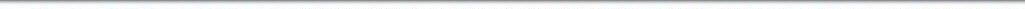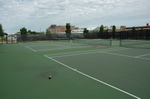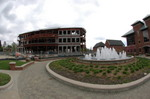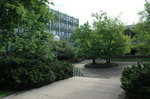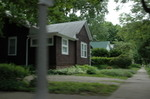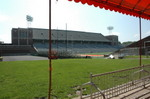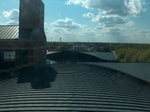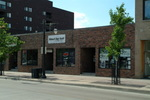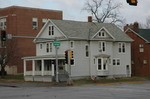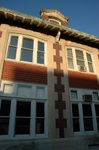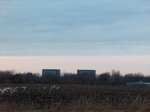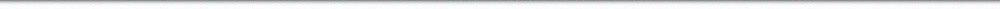N A V I G A T I O N D I G I T A L L I B R A R Y

## Repository: UIHistories Project: Book - Century of Physics (1973) [PAGE 19]

Caption: Book - Century of Physics (1973)
This is a reduced-resolution page image for fast online browsing.

< Previous Page [Displaying Page 19 of 39] Next Page >
[VIEW ALL PAGE THUMBNAILS]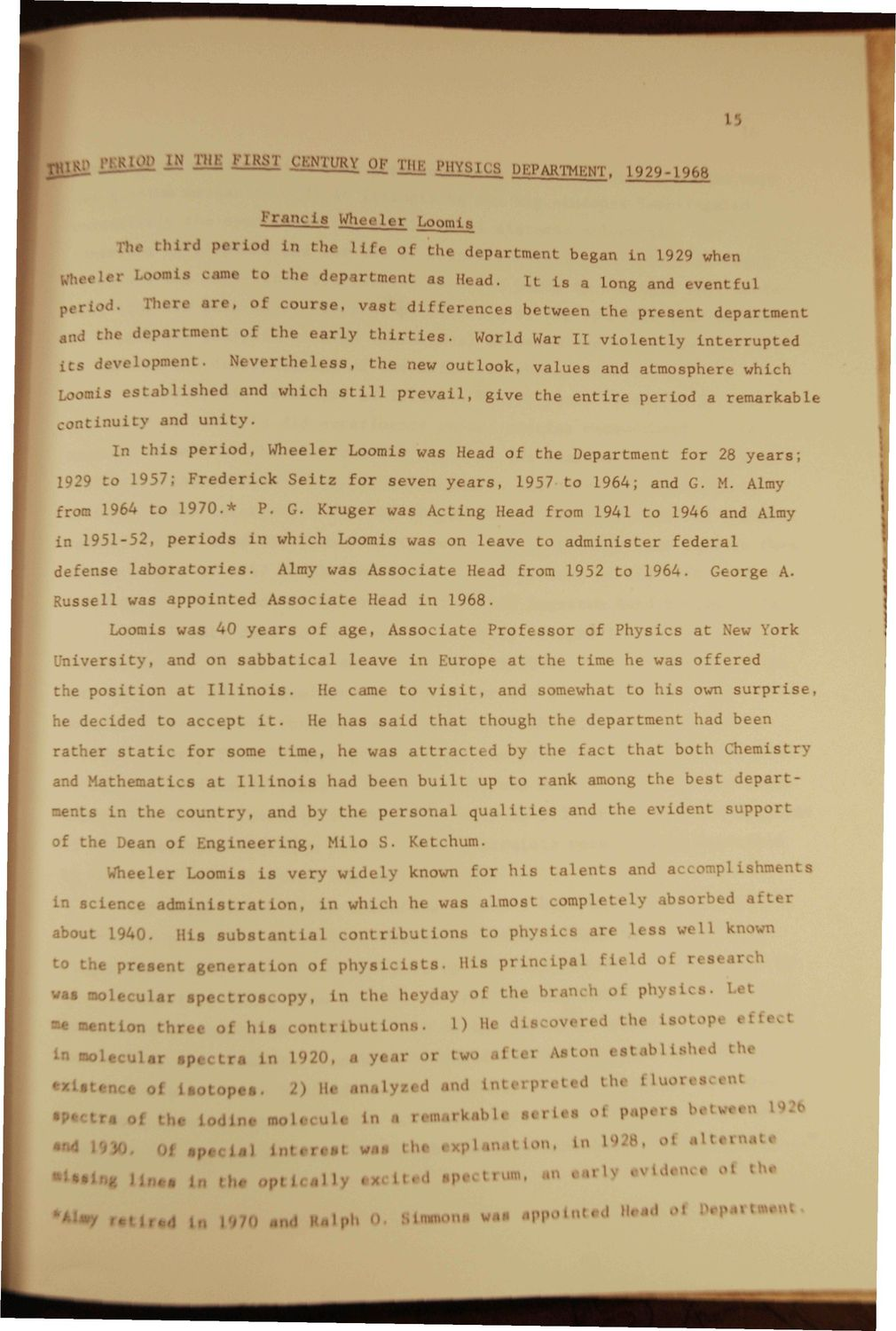### EXTRACTED TEXT FROM PAGE:

1

FIRST J

UKYOK^

PHYSICS

2EARTMENT,

L929-196

F r a n c i s Wheeler Loom is he t h i r d p e r i o d

1,v> ii

Ln t h e

Life oi

th« d e p a r t m e n t began Lfl L929 when It is a long and

n

eventful department interrupted

h e r o « r . . of c o u r s e , v a s t d i f f e r e n c e s b e t * ,.,d t h e depai I t s develO nt oi Ne\ the e a r l y t h i r t i e s . rtheless,

the present

World War I I v i o l e n t l y

t h e new out Look, v a l u e s and atmosphere which prevail, g i v e t h e e n t i r e p e r i o d a remarkable

Loc La e s t a b l i s h e d and which s t i l l c o n t i n u i t y and u n i t y .

In t h i s p e r i o d , W h e e l e r Loomis was Head of t h e Department f o r 28 y e a r s ; 1929 a 1957: F r e d e r i c k S e i t z f o r s e v e n y e a r s , 1957 t o 1964; and G. M. Almy

from 1964 t o 1 9 7 0 . * 1951-5periods

P. G. Kruger was A c t i n g Head from 1941 t o 1946 and Almy i n w h i c h Loomis was on l e a v e t o a d m i n i s t e r federal George A.

defense l a b o r a t o r i e s .

Almy was A s s o c i a t e Head from 1952 t o 1964.

R u s s e l l was a p p o i n t e d A s s o c i a t e Head i n 1968. Loomis was 40 y e a r s of a g e , A s s o c i a t e P r o f e s s o r versit^ he p o s : and on s a b b a t i c a l ion a t Illinois. pt it. of P h y s i c s a t New York offered

l e a v e i n Europe a t t h e time he was to v i s i t ,

He canu

and somewhat t o h i s own s u r p r i s e , though t h e d e p a r t m e n t had been that both Chemistry departsupport

he d e c i d e d t o ace rather s t a t i c for

He h a s s a i d t h a t

some t i m e , he was a t t r a c t e d by t h e f a c t Illinois and b had been b u i l t the personal M i l o S.

and M a t h e m a t i c s a t ments in t h e c o u n t r

up t o I ink among t h e best qualities and t h e e v i d it

of t h e Dean of K n g i n e e i < n g ,

Ketchum. I his .Imost t o pi talents npl 8 .u i pal and ac pi ishmei ar s

Whi- Ler Loomis i s v e r y w i d e l y known n science administrat about 1940. His s u b s t a n t i a l a t i o n oi in wh . h he Wfi contrl tions

a b s o r b e d al L s well < known

t o t h e p r e s e n t g<

phyaiciata.

His p r i i

f i e l d of i» s e a r c h

was molespectrosi >py, L the h day oi th< bran n < phyel i Let «e sent* m • his tti L Loir b 1) He d >< red the iaot * v • «oW lar s era L 1920, a ytar or tv ..ltei Jton eetaM i ad the n t i .. of Leo! pet. lu- •Ml *ed •»<» Ittttn ited the ti tace •P« i ine mol. .U. • ...rWbU l i r l u oi POP" '' "••« *<* oi ^H.. u i Lntoroot was he i Unation, L L928, ol * n ote the * I IOI • ad ili> ••* it Iph II n oori) " *

l

• —

n "•• appointed Heed